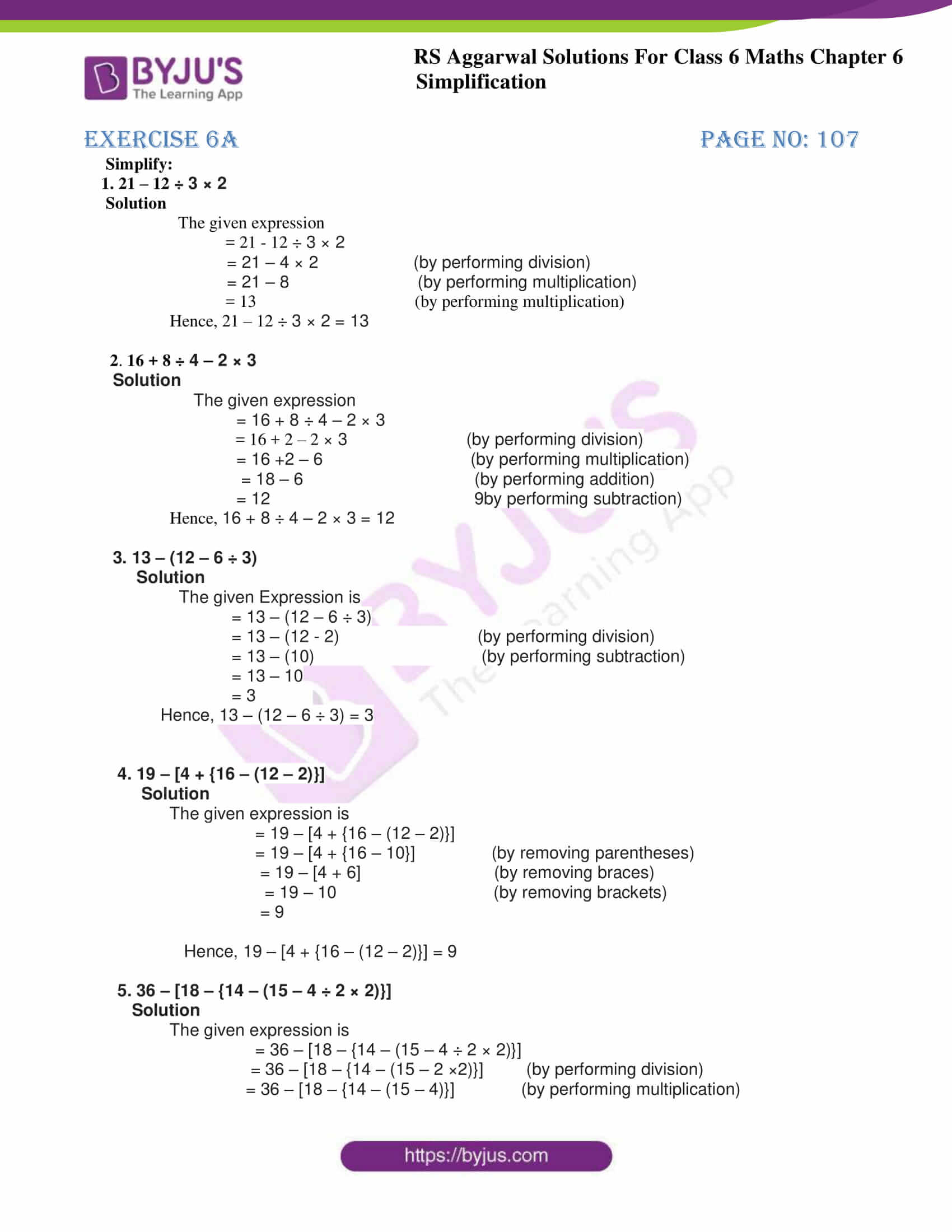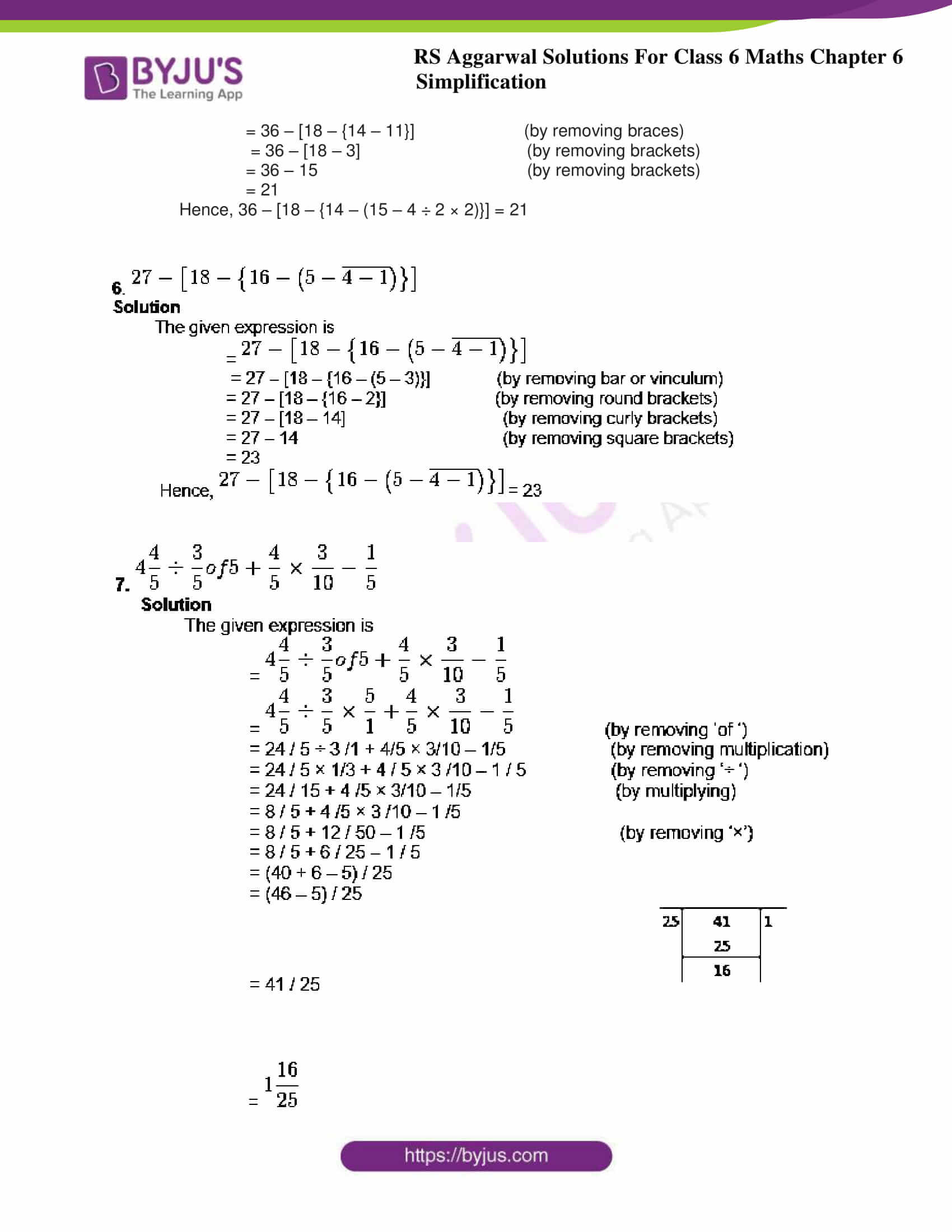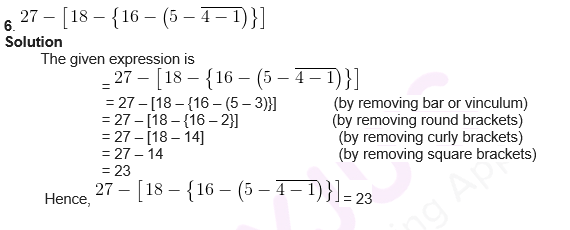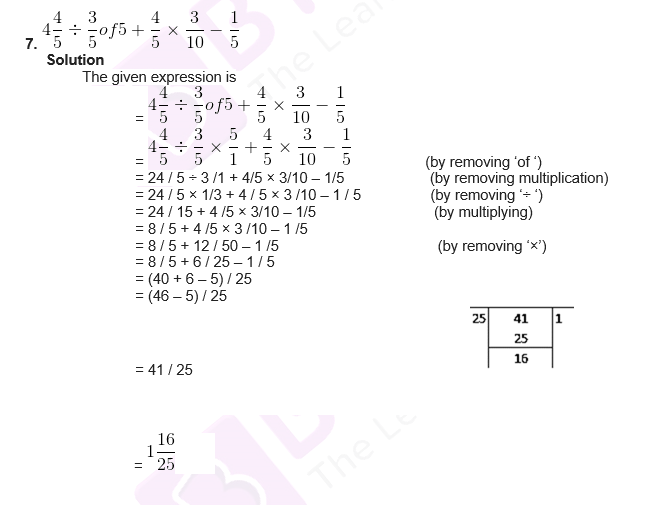# RS Aggarwal Solutions for Class 6 Chapter 6 Simplification Exercise 6A

RS Aggarwal Solutions for Class 6 Chapter 6 Simplification Exercise 6A PDF are available for students to download. Exercise 6A question deals with the Simplification of the given set of numbers using various types of brackets. The expert tutors at BYJU’S in Mathematics prepare solutions in order to help students with their exam preparation and score high marks. Practicing problems using RS Aggarwal Solutions improves logical thinking abilities among students, which is important from the exam point of view.

## Download PDF of RS Aggarwal Solutions for Class 6 Chapter 6 Simplification Exercise 6A### Access answers to Maths RS Aggarwal Solutions for Class 6 Chapter 6 Simplification Exercise 6A

Simplify:

1. 21 – 12 ÷ 3 × 2

Solution

The given expression

= 21 – 12 ÷ 3 × 2

= 21 – 4 × 2 (by performing division)

= 21 – 8 (by performing multiplication)

= 13 (by performing multiplication)

Hence, 21 – 12 ÷ 3 × 2 = 13

2. 16 + 8 ÷ 4 – 2 × 3

Solution

The given expression

= 16 + 8 ÷ 4 – 2 × 3

= 16 + 2 – 2 × 3 (by performing division)

= 16 +2 – 6 (by performing multiplication)

= 18 – 6 (by performing addition)

= 12 9by performing subtraction)

Hence, 16 + 8 ÷ 4 – 2 × 3 = 12

3. 13 – (12 – 6 ÷ 3)

Solution

The given Expression is

= 13 – (12 – 6 ÷ 3)

= 13 – (12 – 2) (by performing division)

= 13 – (10) (by performing subtraction)

= 13 – 10

= 3

Hence, 13 – (12 – 6 ÷ 3) = 3

4. 19 – [4 + {16 – (12 – 2)}]

Solution

The given expression is

= 19 – [4 + {16 – (12 – 2)}]

= 19 – [4 + {16 – 10}] (by removing parentheses)

= 19 – [4 + 6] (by removing braces)

= 19 – 10 (by removing brackets)

= 9

Hence, 19 – [4 + {16 – (12 – 2)}] = 9

5. 36 – [18 – {14 – (15 – 4 ÷ 2 × 2)}]

Solution

The given expression is

= 36 – [18 – {14 – (15 – 4 ÷ 2 × 2)}]

= 36 – [18 – {14 – (15 – 2 ×2)}] (by performing division)

= 36 – [18 – {14 – (15 – 4)}] (by performing multiplication)

= 36 – [18 – {14 – 11}] (by removing braces)

= 36 – [18 – 3] (by removing brackets)

= 36 – 15 (by removing brackets)

= 21

Hence, 36 – [18 – {14 – (15 – 4 ÷ 2 × 2)}] = 21